# Explain Various Types of High Pass Filters

## High Pass Filter

A High Pass Filter or HPF, is the exact opposite to that of the previously discussed Low Pass filter circuit .

The passive high pass filter circuit as its name implies, only passes signals above the selected cut-off point, ƒc eliminating any low frequency signals from the waveform, where as the low pass filter only allows signals to pass below its cut-off frequency point, ƒc,

The high pass filters are also of R-C, R-L, inverted L, T-type and π-types .

### High Pass R-C Filter Circuit

The high pass R-C filter circuit is shown in fig. below.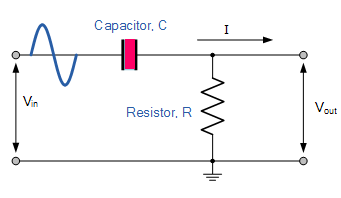In this circuit the output voltage is taken across the resistor R.

The reactance of the capacitor C is given as :That means the reactance of the capacitor decreases with increase in frequency.

Hence at low frequencies the capacitor C offers considerable reactance and so blocks them. But, at higher frequencies it offers little reactance and allows them to pass through it.

The output voltage is developed across the resistor R.

Since the circuit blocks the low frequencies but allows high frequency signals to pass through it, the circuit is called high pass R-C circuit.

With increase in frequency, the reactance of the capacitor decreases and therefore, the output and gain increases.

At very high frequencies, the capacitive reacatnce becomes very small, so the output becomes almost equal to the input and gain becomes equal to unity.

### Frequency Response of  High Pass Filter

The frequency response curve for the high pass filter is shown in fig. below.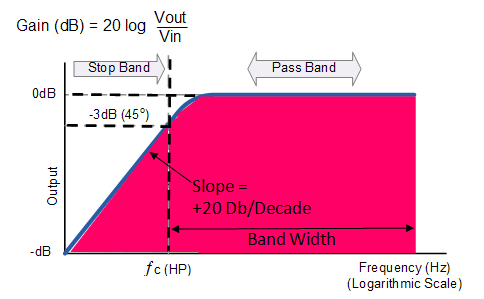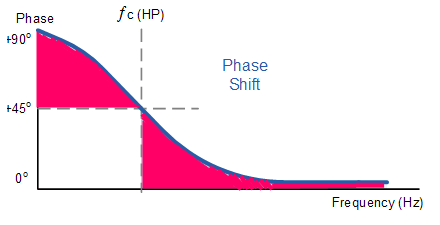The Bode Plot or Frequency Response Curve above for a passive high pass filter is the exact opposite to that of a low pass filter.

Here the signal is attenuated or blocked at low frequencies with the output increasing at +20dB/Decade (6dB/Octave) until the frequency reaches the cut-off point ( ƒc ) where again R = Xc.

It has a response curve that extends down from infinity to the cut-off frequency, where the output voltage amplitude is 1/√2  = 70.7% of the input signal value or -3dB (20 log (Vout/Vin)) of the input value.

Also we can see that the phase angle ( Φ ) of the output signal leads that of the input and is equal to +45o at frequency ƒc.

Hence, at cutoff frequency, R = X, the phase angle is 45and output power is half of the input power. The output voltage is 0.707 times the maximum value of voltage  Vmax .

The frequency response curve for this filter implies that the filter can pass all signals out to infinity. However in practice, the filter response does not extend to infinity but is limited by the electrical characteristics of the components used.

The cut-off frequency point for a high pass filter can be found using the same equation as that of the low pass filter, as shown below.

#### Cutoff frequency of High Pass Filter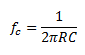### High Pass R-L Filter Circuit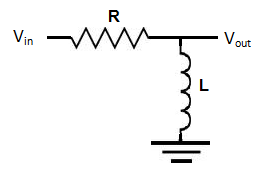In such a filter the resistance R offers fixed opposition.

However,  the reactance offered by the inductor L increases with the increase in frequency, so high frequency signal develops across L but signal below cutoff frequency fc develops negligible voltage across inductor L .

High frequency output voltage Vout developed across inductor is given as :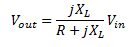and the cutoff frequency is given as :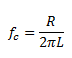### Inverted L-type High Pass Filter Circuit

The circuit of an inverted L-type High pass filter is shown below.At low frequencies, the reactance offered by the capacitor is large but reactance offered by the inductor L is small, hence output voltage developed across inductor L is very small.

But at higher frequencies Xbecomes much larger and Xis reduced so considerable output voltage is developed across L.

Thus lower frequencies are blocked and higher frequencies are passed.

### T-type High Pass Filter

The circuit of a T-type High pass filter is shown below.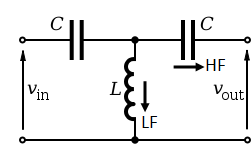This circuit employs an additional capacitor as shown in fig. above, to improve the filtering action.

### π-type High Pass Filter

Such a filter circuit employs one capacitor and two inductors  as shown in fig. below.The inductors shunt out the lower frequencies.

It can be noted that in such filters that capacitors are connected in series between the input and output and the inductors are grounded in parallel.

The output voltage is taken across the inductor.